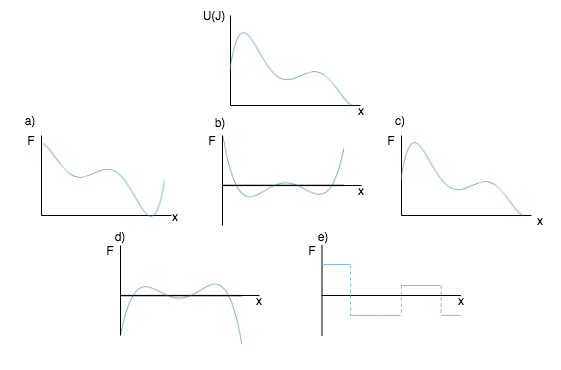# Potential Energy to Force#

## Part 1#

The potential energy of a particle in a conservative one-dimensional potential as a function of position, $$x$$, is shown. Which figure represents the force as a function of position $$x$$ that acts on the particle?• Figure A

• Figure B

• Figure C

• Figure D

• Figure E